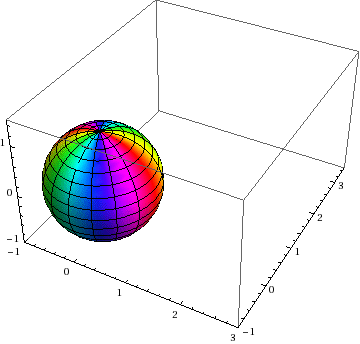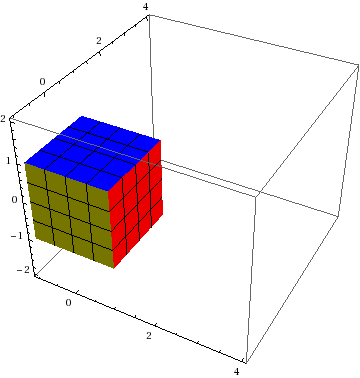# Tutorial: Visualizing Deformation

If you’ve never heard of a continuum mapping, read our introduction to mappings.

This posting discusses the two most common visualization methods for 3D homogeneous mappings: Showing how a sphere transforms to an ellipsoid and how a cube transforms to a parallelepiped:The deformed ellipsoid is sometimes called a Reynolds glyph (though this term sometimes means different things to different people).

For each$\vec{X}$ in the initial configuration (sphere or cube), you apply the mapping to obtain the corresponding$\vec{x}$ in the deformed configuration. The motion depicted here is homogeneous, meaning that it is affine with respect to$\vec{X}$, which means that$\vec{x}(\vec{X},t)=\bold{F}(t)\cdot\vec{X}+\vec{c}(t)$, where$\bold{F}(t)$ is the time-varying (but not position dependent) deformation gradient tensor and$\vec{c}(t)$ is the time-varying (but not position dependent) displacement of the point that was initially at the origin. For a homogeneous deformation, the time dependence might be nonlinear, but the one depicted here happens to be affine in time, resulting in constant particle velocity (i.e., each particle in the body follows its own straight line trajectory with no change in speed).

For non-homogeneous mappings, spheres no longer deform to ellipsoids, nor do cubes deform to parallelepipeds. In that case, though, these concepts still apply to infinitesimal volumes. This statement is analogous to the assertion that knowing the properties of straight lines allows you, through calculus, to make statements about curved lines — that’s because curves are regarded informally as an infinite collection of infinitesimal straight line segments.

If you have Mathematica, you can download the notebook file used to create the above animations here.

AUTHOR NOTE: I would appreciate someone helping me to learn how to post stuff like this more elegantly using Wolfram’s CDF format.